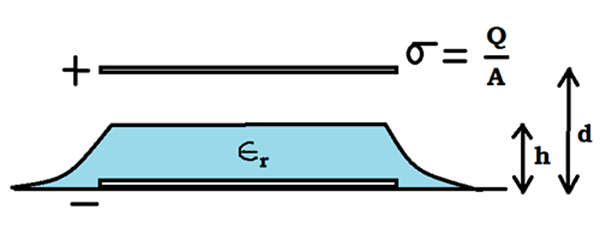The Wet Condenser?!A Parallel Plate Condenser is charged with a surface charge density $\displaystyle\sigma = \frac{Q}{A}$. Due to a pipe leakage, water flows in. It is observed that the water surface rises to a height $h$ above the bottom plate.

Find the height $h$ (in cm) to which the water level rises.

Details and Assumptions:
$\bullet$ $\epsilon_r = 80$
$\bullet$ $\rho_{water} = 1000 Kg/m^3$
$\bullet$ $g = 9.8 m/s^2$
$\bullet$ $\sigma = 2 \mu C/m^2$

×